Publicité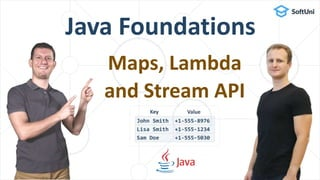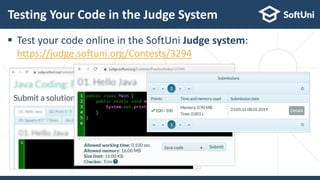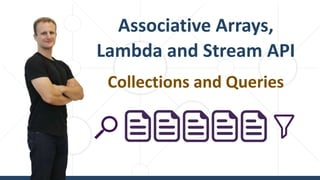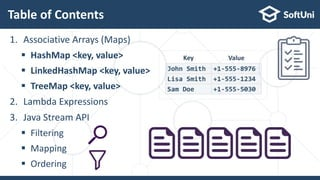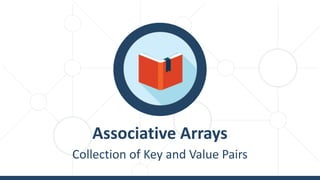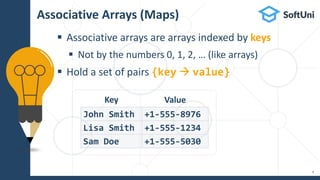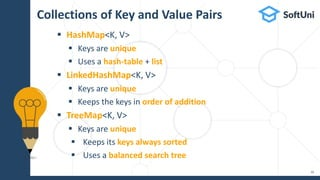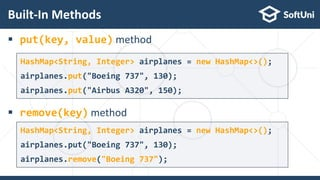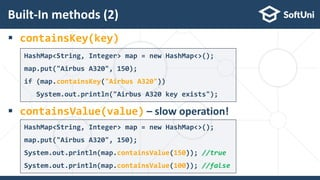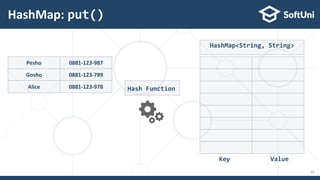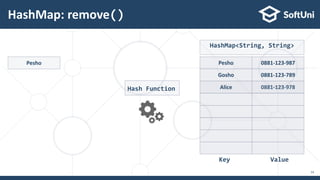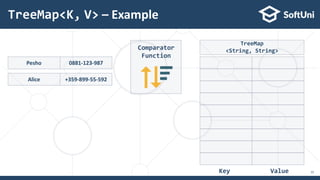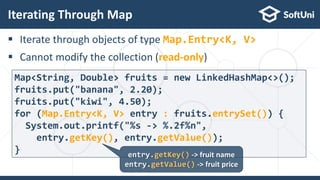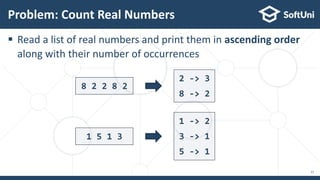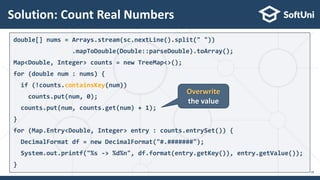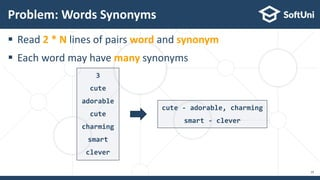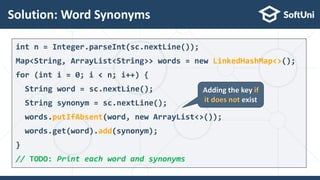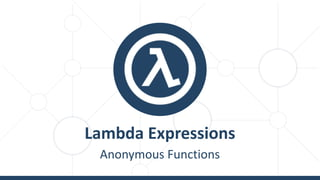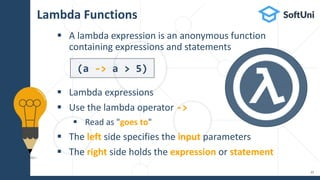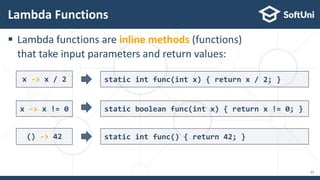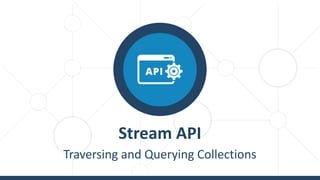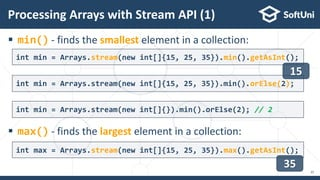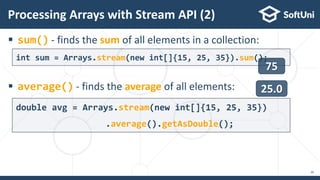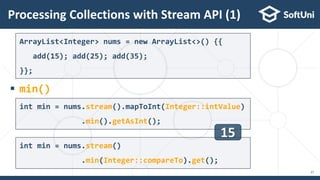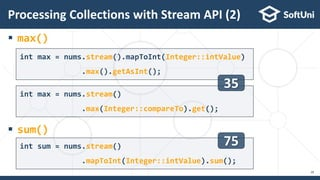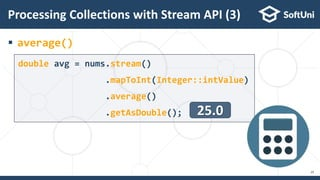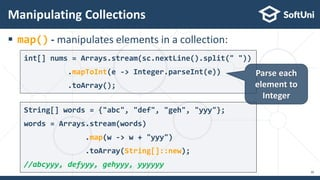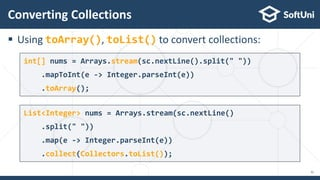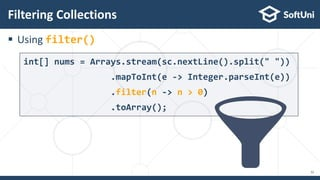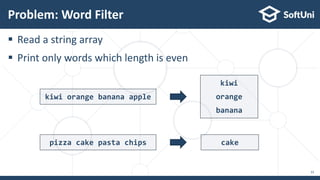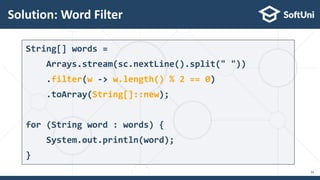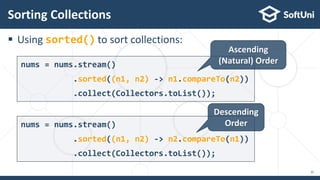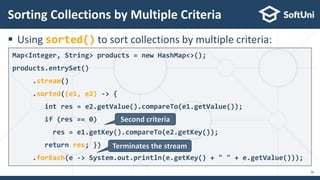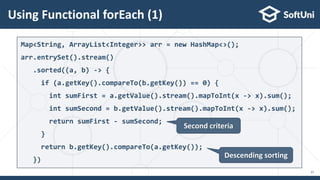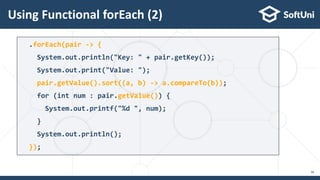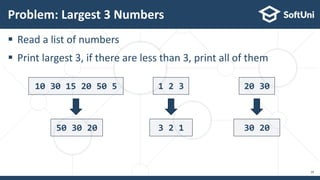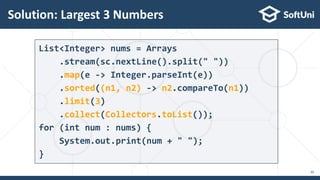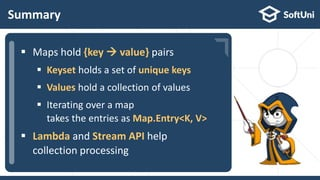1 sur 41
Publicité

### Java Foundations: Maps, Lambda and Stream API

1. Java Foundations Maps, Lambda and Stream API
2. Your Course Instructors Svetlin Nakov George Georgiev
3. The Judge System Sending your Solutions for Automated Evaluation
4. Testing Your Code in the Judge System  Test your code online in the SoftUni Judge system: https://judge.softuni.org/Contests/3294
5. Associative Arrays, Lambda and Stream API Collections and Queries
6. Table of Contents 1. Associative Arrays (Maps)  HashMap <key, value>  LinkedHashMap <key, value>  TreeMap <key, value> 2. Lambda Expressions 3. Java Stream API  Filtering  Mapping  Ordering
7. Associative Arrays Collection of Key and Value Pairs
8.  Associative arrays are arrays indexed by keys  Not by the numbers 0, 1, 2, … (like arrays)  Hold a set of pairs {key  value} Associative Arrays (Maps) 9 John Smith +1-555-8976 Lisa Smith +1-555-1234 Sam Doe +1-555-5030 Key Value
9. Collections of Key and Value Pairs  HashMap<K, V>  Keys are unique  Uses a hash-table + list  LinkedHashMap<K, V>  Keys are unique  Keeps the keys in order of addition  TreeMap<K, V>  Keys are unique  Keeps its keys always sorted  Uses a balanced search tree 10
10.  put(key, value) method  remove(key) method Built-In Methods 11 HashMap<String, Integer> airplanes = new HashMap<>(); airplanes.put("Boeing 737", 130); airplanes.remove("Boeing 737"); HashMap<String, Integer> airplanes = new HashMap<>(); airplanes.put("Boeing 737", 130); airplanes.put("Airbus A320", 150);
11.  containsKey(key)  containsValue(value) – slow operation! Built-In methods (2) 12 HashMap<String, Integer> map = new HashMap<>(); map.put("Airbus A320", 150); System.out.println(map.containsValue(150)); //true System.out.println(map.containsValue(100)); //false HashMap<String, Integer> map = new HashMap<>(); map.put("Airbus A320", 150); if (map.containsKey("Airbus A320")) System.out.println("Airbus A320 key exists");
12. HashMap: put() 13 HashMap<String, String> Key Value Hash Function Pesho 0881-123-987 Gosho 0881-123-789 Alice 0881-123-978
13. HashMap: remove() 14 HashMap<String, String> Key Value Pesho Pesho Gosho 0881-123-987 0881-123-789 Alice 0881-123-978 Hash Function
14. Pesho 0881-123-987 TreeMap<K, V> – Example 15 TreeMap <String, String> Key Value Alice +359-899-55-592 Comparator Function
15. Map<String, Double> fruits = new LinkedHashMap<>(); fruits.put("banana", 2.20); fruits.put("kiwi", 4.50); for (Map.Entry<K, V> entry : fruits.entrySet()) { System.out.printf("%s -> %.2f%n", entry.getKey(), entry.getValue()); }  Iterate through objects of type Map.Entry<K, V>  Cannot modify the collection (read-only) Iterating Through Map 16 entry.getKey() -> fruit name entry.getValue() -> fruit price
16.  Read a list of real numbers and print them in ascending order along with their number of occurrences Problem: Count Real Numbers 17 8 2 2 8 2 2 -> 3 8 -> 2 1 5 1 3 1 -> 2 3 -> 1 5 -> 1
17. Solution: Count Real Numbers 18 double[] nums = Arrays.stream(sc.nextLine().split(" ")) .mapToDouble(Double::parseDouble).toArray(); Map<Double, Integer> counts = new TreeMap<>(); for (double num : nums) { if (!counts.containsKey(num)) counts.put(num, 0); counts.put(num, counts.get(num) + 1); } for (Map.Entry<Double, Integer> entry : counts.entrySet()) { DecimalFormat df = new DecimalFormat("#.#######"); System.out.printf("%s -> %d%n", df.format(entry.getKey()), entry.getValue()); } Overwrite the value
18.  Read 2 * N lines of pairs word and synonym  Each word may have many synonyms Problem: Words Synonyms 19 3 cute adorable cute charming smart clever cute - adorable, charming smart - clever
19. Solution: Word Synonyms 20 int n = Integer.parseInt(sc.nextLine()); Map<String, ArrayList<String>> words = new LinkedHashMap<>(); for (int i = 0; i < n; i++) { String word = sc.nextLine(); String synonym = sc.nextLine(); words.putIfAbsent(word, new ArrayList<>()); words.get(word).add(synonym); } // TODO: Print each word and synonyms Adding the key if it does not exist
20. Lambda Expressions Anonymous Functions
21. Lambda Functions  A lambda expression is an anonymous function containing expressions and statements  Lambda expressions  Use the lambda operator ->  Read as "goes to"  The left side specifies the input parameters  The right side holds the expression or statement 22 (a -> a > 5)
22.  Lambda functions are inline methods (functions) that take input parameters and return values: Lambda Functions 23 x -> x / 2 static int func(int x) { return x / 2; } static boolean func(int x) { return x != 0; } x -> x != 0 () -> 42 static int func() { return 42; }
23. Stream API Traversing and Querying Collections
24.  min() - finds the smallest element in a collection:  max() - finds the largest element in a collection: Processing Arrays with Stream API (1) 25 int min = Arrays.stream(new int[]{15, 25, 35}).min().getAsInt(); int max = Arrays.stream(new int[]{15, 25, 35}).max().getAsInt(); 35 int min = Arrays.stream(new int[]{15, 25, 35}).min().orElse(2); int min = Arrays.stream(new int[]{}).min().orElse(2); // 2 15
25.  sum() - finds the sum of all elements in a collection:  average() - finds the average of all elements: Processing Arrays with Stream API (2) 26 int sum = Arrays.stream(new int[]{15, 25, 35}).sum(); double avg = Arrays.stream(new int[]{15, 25, 35}) .average().getAsDouble(); 75 25.0
26.  min() Processing Collections with Stream API (1) 27 ArrayList<Integer> nums = new ArrayList<>() {{ add(15); add(25); add(35); }}; int min = nums.stream().mapToInt(Integer::intValue) .min().getAsInt(); int min = nums.stream() .min(Integer::compareTo).get(); 15
27.  max()  sum() Processing Collections with Stream API (2) 28 int max = nums.stream().mapToInt(Integer::intValue) .max().getAsInt(); int max = nums.stream() .max(Integer::compareTo).get(); int sum = nums.stream() .mapToInt(Integer::intValue).sum(); 35 75
28.  average() Processing Collections with Stream API (3) 29 double avg = nums.stream() .mapToInt(Integer::intValue) .average() .getAsDouble(); 25.0
29.  map() - manipulates elements in a collection: Manipulating Collections 30 String[] words = {"abc", "def", "geh", "yyy"}; words = Arrays.stream(words) .map(w -> w + "yyy") .toArray(String[]::new); //abcyyy, defyyy, gehyyy, yyyyyy int[] nums = Arrays.stream(sc.nextLine().split(" ")) .mapToInt(e -> Integer.parseInt(e)) .toArray(); Parse each element to Integer
30.  Using toArray(), toList() to convert collections: Converting Collections 31 int[] nums = Arrays.stream(sc.nextLine().split(" ")) .mapToInt(e -> Integer.parseInt(e)) .toArray(); List<Integer> nums = Arrays.stream(sc.nextLine() .split(" ")) .map(e -> Integer.parseInt(e)) .collect(Collectors.toList());
31.  Using filter() Filtering Collections 32 int[] nums = Arrays.stream(sc.nextLine().split(" ")) .mapToInt(e -> Integer.parseInt(e)) .filter(n -> n > 0) .toArray();
32.  Read a string array  Print only words which length is even Problem: Word Filter 33 kiwi orange banana apple kiwi orange banana pizza cake pasta chips cake
33. Solution: Word Filter 34 String[] words = Arrays.stream(sc.nextLine().split(" ")) .filter(w -> w.length() % 2 == 0) .toArray(String[]::new); for (String word : words) { System.out.println(word); }
34.  Using sorted() to sort collections: Sorting Collections 35 nums = nums.stream() .sorted((n1, n2) -> n1.compareTo(n2)) .collect(Collectors.toList()); nums = nums.stream() .sorted((n1, n2) -> n2.compareTo(n1)) .collect(Collectors.toList()); Ascending (Natural) Order Descending Order
35.  Using sorted() to sort collections by multiple criteria: Sorting Collections by Multiple Criteria 36 Map<Integer, String> products = new HashMap<>(); products.entrySet() .stream() .sorted((e1, e2) -> { int res = e2.getValue().compareTo(e1.getValue()); if (res == 0) res = e1.getKey().compareTo(e2.getKey()); return res; }) .forEach(e -> System.out.println(e.getKey() + " " + e.getValue())); Second criteria Terminates the stream
36. Using Functional forEach (1) 37 Map<String, ArrayList<Integer>> arr = new HashMap<>(); arr.entrySet().stream() .sorted((a, b) -> { if (a.getKey().compareTo(b.getKey()) == 0) { int sumFirst = a.getValue().stream().mapToInt(x -> x).sum(); int sumSecond = b.getValue().stream().mapToInt(x -> x).sum(); return sumFirst - sumSecond; } return b.getKey().compareTo(a.getKey()); }) Second criteria Descending sorting
37. Using Functional forEach (2) 38 .forEach(pair -> { System.out.println("Key: " + pair.getKey()); System.out.print("Value: "); pair.getValue().sort((a, b) -> a.compareTo(b)); for (int num : pair.getValue()) { System.out.printf("%d ", num); } System.out.println(); });
38.  Read a list of numbers  Print largest 3, if there are less than 3, print all of them Problem: Largest 3 Numbers 39 10 30 15 20 50 5 50 30 20 1 2 3 3 2 1 20 30 30 20
39. Solution: Largest 3 Numbers 40 List<Integer> nums = Arrays .stream(sc.nextLine().split(" ")) .map(e -> Integer.parseInt(e)) .sorted((n1, n2) -> n2.compareTo(n1)) .limit(3) .collect(Collectors.toList()); for (int num : nums) { System.out.print(num + " "); }
40.  …  …  … Summary  Maps hold {key  value} pairs  Keyset holds a set of unique keys  Values hold a collection of values  Iterating over a map takes the entries as Map.Entry<K, V>  Lambda and Stream API help collection processing
41.  …  …  … Next Steps  Join the SoftUni "Learn To Code" Community  Access the Free Coding Lessons  Get Help from the Mentors  Meet the Other Learners https://softuni.org

### Notes de l'éditeur

1. Hello, I am Svetlin Nakov from SoftUni (the Software University). Together with my colleague George Georgiev, we continue teaching this free Java Foundations course, which covers important concepts from Java programming, such as arrays, lists, maps, methods, strings, classes, objects and exceptions, and prepares you for the "Java Foundations" official exam from Oracle. In this lesson your instructor George will explain the concept of associative arrays (or maps), which hold key-to-value mappings. He will demonstrate through live code examples how to use standard API classes, such as Map, HashMap and TreeMap, to work with maps in Java. Just after the maps, the instructor will explain the concept of lambda expressions and how to define and use lambda functions in Java through the arrow operator. Lambda expressions are important in Java programming, because they enable processing and querying Java collections, using the Java Stream API. In this lesson, the instructor will demonstrate the power of the Stream API, and how to map, filter and sort sequences of elements (such as arrays, lists and maps), how to extract subsequences, how to convert a sequence to array or list and many other operations over sequences. Let's learn maps, lambda functions and the Stream API in Java through live coding examples, and later solve some hands-on exercises to gain practical experience. Are you ready? Let's start!
2. Before the start, I would like to introduce your course instructors: Svetlin Nakov and George Georgiev, who are experienced Java developers, senior software engineers and inspirational tech trainers. They have spent thousands of hours teaching programming and software technologies and are top trainers from SoftUni. I am sure you will like how they teach programming.
3. Most of this course will be taught by George Georgiev, who is a senior software engineer with many years of experience with Java, JavaScript and C++. George enjoys teaching programming very much and is one of the top trainers at the Software University, having delivered over 300 technical training sessions on the topics of data structures and algorithms, Java essentials, Java fundamentals, C++ programming, C# development and many others. I have no doubt you will benefit greatly from his lessons, as he always does his best to explain the most challenging concepts in a simple and fun way.
4. Before we dive into the course, I want to show you the SoftUni judge system, where you can get instant feedback for your exercise solutions. SoftUni Judge is an automated system for code evaluation. You just send your code for a certain coding problem and the system will tell you whether your solution is correct or not and what exactly is missing or wrong. I am sure you will love the judge system, once you start using it!
5. // Solution to problem "01. Student Information". import java.util.Scanner; public class Main { public static void main(String[] args) { Scanner sc = new Scanner(System.in); String name = sc.nextLine(); int age = Integer.parseInt(sc.nextLine()); double grade = Double.parseDouble(sc.nextLine()); System.out.printf("Name: %s, Age: %d, Grade: %.2f", name, age, grade); } }
6. Now it’s time to start the lesson. Your instructor George will explain the concept of maps in Java and how to use the HashMap and TreeMap classes. He will teach you how to use lambda expressions and the Java Stream API to map, filter and sort lists, arrays and other collections. Let’s start.